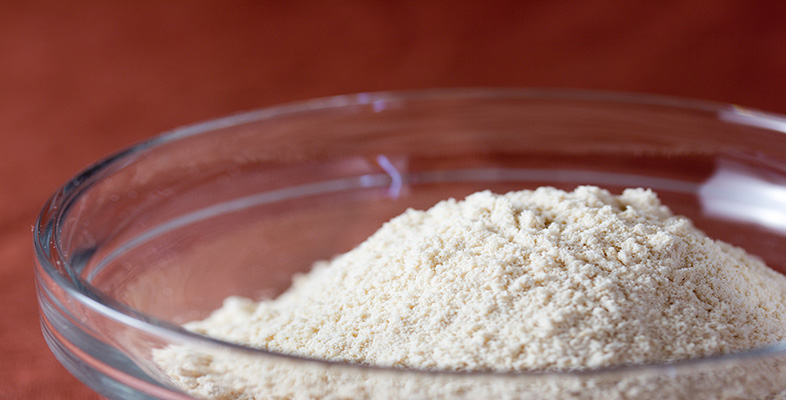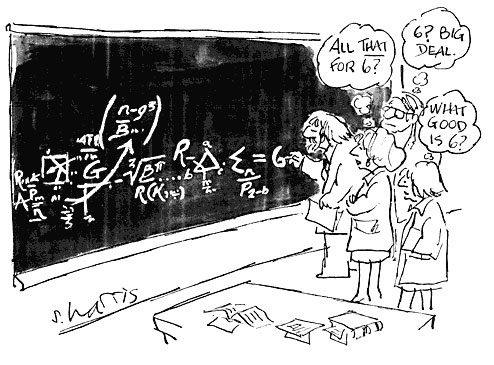Rounding and estimation

This free course is available to start right now. Review the full course description and key learning outcomes and create an account and enrol if you want a free statement of participation.

Free course

1.5 Significant figures

Sometimes it doesn’t make sense to round to a specific number of decimal places. If, say, you were calculating the cost of fencing at £10.65 per metre, for a garden boundary, the length of which had been given to you as 185 feet, then you would want to multiply 10.65 × 185 × 0.3048. (Conversion of feet to metres was given in Example 2.) To do the calculation quickly, you might round this to 10 × 200 × 0.3 = 600 and thus estimate the cost as about £600. (You might use this figure as a check on the exact calculation, done on a calculator.)What has been done is round each of 10.65, 185, 0.3048 to one significant figure. (We often write ‘s.f.’ for ‘significant figures’.) Thus 10.65 is rounded to 10 (the 1 is the significant figure); 185 has been rounded to 200 (the 2 is the significant figure); and 0.3048 has been rounded to 0.3 (the 3 is the significant figure). All of these numbers contained zeros, but they were not deemed significant so far as the accuracy of the estimation was concerned. In the £600 answer, only the 6 is significant. You would expect the answer to be about six hundreds but not zero tens and zero units.

The first non zero digit (from the left) in a number is the first significant figure. To round to a given number of significant figures, first count from the first significant digit to the number required (including zeros). If the next digit is 5, 6, 7, 8 or 9 round up, otherwise round down.

So that your readers know how a number has been rounded, it is important to state the number of significant figures.

For example, if you round 475 to 1 significant figure, you can write 475 = 500 (to 1 s.f.).

When a number is rounded, the number of significant figures is known as the precision of the number.

Example 3

A particular mobile phone allows you to check the free space available in the memory. Suppose the display shows 2354 856 bytes. Express this available memory space correct to:

• (a) One significant figure (1 s.f.), which might be appropriate if comparing it to another phone.

• (b) Two significant figures (2 s.f.), which might be appropriate if working out if there is enough memory for a large picture file.

• (a) One significant figure: the first digit (from the left) is the 2. The next significant digit is the 3, and since this is less than 5 round down. Thus the available memory space in the phone is about 2000 000 bytes (to 1 s.f.).

• (b) Two significant figures: the third significant digit is the 5, so round up. Thus the available memory space in the phone is about 2400 000 bytes (to 2 s.f.).

MU120_4M2